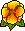## Crack singularities for general elliptic systems

Martin Costabel, Monique DaugeWe consider general homogeneous Agmon-Douglis-Nirenberg elliptic systems with constant coefficients complemented by the same set of boundary conditions on both sides of a crack in a two-dimensional domain. We prove that the singular functions all behave as half-integer powers of the distance r to the crack tip, with the possible exception of a finite number of singularities behaving as the product of an integer power of r and a logarithm of r. We also prove results about singularities in the case when the boundary conditions on the two sides of the crack are not the same, and in particular in mixed Dirichlet-Neumann boundary value problems for strongly coercive systems: in the latter case, we prove that the real part of the exponents of singularity have the form 1/4 + k/2 with integer k. This is valid for general anisotropic elasticity too.Dec. 1999. Preprint IRMAR 00-14.

Published in Math. Nach. 235, 29-49 (2002).Fichier pdf